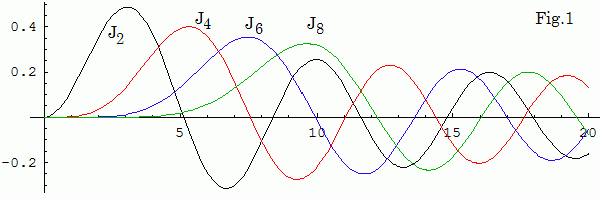ω γ Xo Yo delay it
framerate

Use your fingers or mouse to control the model (hold shift key or use mouse wheel to zoom it). Canvas is matched to your browser window. 0 < Xo,Yo < 280. The script makes 2 it iterations with the time steps Δt = 0.05 per frame. Set it = 1 and γ = 0 after you get a stationary pattern.

# Resonances in circular membrane

A CPU based simulation of the wave equation with time periodic perturbation. For this membrane wave eigenmodes are
un,ω(r,φ,t) = Jn(ωr) sin(nφ) sin(ωt),
where Jn are the Bessel functions (J2,4,6,8 are shown in Fig.1). From the boundary condition un,ω(r = 1) = 0 it follows thet ω are zeros of the Bessel functions. Some of them you can find below.
ω2 = {5.136, 8.417, 11.62, 14.80, 17.96}
ω4 = {7.588, 11.06, 14.37, 17.62, 20.83}
ω6 = {9.936, 13.59, 17.00, 20.32, 23.59}
ω8 = {12.22, 16.04, 19.55, 22.95, 26.27}
ω10 = {14.48, 18.43, 22.05, 25.51, 28.89}
ω12 = {16.70, 20.79, 24.49, 28.03, 31.46}WebGL Demos     updated 7 August 2010